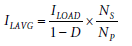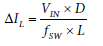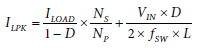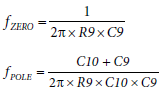Оценочные платы \$86.90## Ресурсы проектирования

### Файлы проектирования и интеграции

• Schematic
• Bill of Materials
• Gerber Files
• Layout Files (Allegro)
• Assembly Drawing Загрузить файлы проектов 328.1 K

### Оценочные платы

Буква "Z" в наименовании компонента указывает на соответствие требованиям RoHS. Отмеченные платы нужны для оценки данной схемы

• EVAL-CN0342-EB1Z (\$86.90) Flyback Power Supply Using a High Stability Isolated Error Amplifier

## Особенности и преимущества

• Isolated flyback power supply
• Linear isolated error amplifier
• Linear isolated error amplifier

## Функции и преимущества схемы

The circuit shown in Figure 1 is an isolated, flyback power supply that uses a linear isolated error amplifier to supply the feedback signal from the secondary side to the primary side. Unlike optocoupler-based solutions, which have a nonlinear transfer function that changes over time and temperature, the linear transfer function of the isolated amplifier is stable and minimizes offset and gain errors when transferring the feedback signal across the isolation barrier.

The entire circuit operates from 5 V to 24 V, allowing it to be used with standard industrial and automotive power supplies. The output capability of the circuit is up to 1 A with a 5 V input and 5 V output configuration.

This solution can be adapted for use in applications where higher dc input voltages are used to create lower voltage isolated supplies with good efficiency and a small form factor. Examples include 10 W to 20 W telecommunication and server power supplies, where power efficiency and printed circuit board (PCB) density are important, and −48 V supplies are common.

## Описание схемы

The isolation amplifier is the ADuM3190, which includes a 1.225 V voltage reference and an error amplifier with 10 MHz unity-gain bandwidth product. An external resistive divider (R1, R2, R3, and R4) and compensation network (R9, C9, and C10) complete the analog feedback loop.

The input supply range of the ADuM3190 is 3.0 V to 20 V on both sides, and internal low dropout regulators provide stable supplies for the voltage reference, error amplifier, and analog isolator. The ADuM3190 is compatible with the Distributed-power Open Standards Alliance (DOSA) output voltage trim method.

The ADP1621 provides pulse-width modulation (PWM) control for the flyback power supply. The internal 5.5 V shunt regulator provides the capability of high supply input voltage with the addition of an external NPN transistor (Q2). The ADP1621 also provides lossless current sensing for current mode operation, providing excellent line and load transient response.

### Setting the Output Voltage

The output voltage is set through a voltage divider from VOUT to the −IN pin of ADuM3190. The feedback resistor ratio sets the output voltage of the system. Using the internal voltage reference in the ADuM3190, the regulation voltage at the −IN pin is 1.225 V.For a 5 V output configuration, the resistor divider values are R1 = 2 kΩ, R2 = 47 kΩ, R3 = 51 kΩ, and R4 = 100 kΩ.

### Voltage Reference

The ADuM3190 provides an internal 1.225 V voltage reference specified for ±1% accuracy over the −40°C to +125°C temperature range. The reference voltage output pin (REFOUT) can be connected to the +IN pin of the error amplifier to set the output voltage. When higher accuracy or a special output voltage is required, and a different reference voltage must be used, the +IN pin can also be connected to an external reference.

### Transformer Selection

The transformer choice is important because it dictates the primary inductor current ripple.

For this design example, the following parameters were used:

• VIN = 5 V
• VOUT = 5 V
• IOUTMAX = 1 A
• fSW = 200 kHz
• Transformer turn ratio = 1:1

The average transformer primary side current, ILAVG, is given bywhere:

D is the duty cycle under maximum load current.
NS/NP is the turn ratio of the transformer.

The output voltage isThe duty cycle (D) is 50% because the output and input are both 5 V, and the transformer turn ratio is 1:1.

The peak-to-peak primary inductor ripple current is inversely proportional to the inductor value.where:

fSW is the switching frequency.
L is the primary inductor value.

Assuming continuous conduction mode (CCM) operation, the primary inductor current is given byAssuming the primary ripple current is 50% of the average current on the transformer primary side, a reasonable choice for the inductor value isThe transformer used in this design is a 1:1 turn ratio transformer with 16 μH primary side inductance (Halo Electronics, Inc., TGB01-P099EP13LF).

### Compensation Network

In a flyback topology power supply, the output load resistance, the output capacitor, and its effective series resistance (ESR) add a zero and a pole at frequencies that are dependent on the component type and values. There is also a right half plane (RHP) zero in the control-to-output transfer function. Because the RHP zero reduces the phase by 90°, the frequency of 0 dB gain (crossover frequency) is lower than the RHP zero.

With the ADuM3190 providing the error amplifier, a Type II compensation network can be provided from the −IN pin to the COMP pin to compensate for the control loop for stability. The compensation network values depend on the selected components.

The zeros and poles of a Type II compensation network are derived as follows:In this particular design, the compensation network is set by R9 = 15 kΩ, C9 = 2.2 nF, and C10 = 1 nF

The zero and the pole provided by this compensation network are fZERO = 4.8 kHz and fPOLE = 15.4 kHz. Increasing the zero and pole frequency improves the load transient response but decreases the phase margin of the feedback loop and can cause instability in the power supply.

### Snubber Network

When the power MOSFET (Q3) is turned off, there is a high voltage spike on the drain due to the transformer leakage inductance. This excessive voltage can overstress the power MOSFET and lead to a reliability issue or damage. Therefore, it is necessary to use an additional network to clamp the voltage.

The resistor, capacitor, and diode (R19, C21, and D2) snubber network absorbs the current in the leakage inductance by turning on the snubber diode when the MOSFET drain voltage exceeds the cathode voltage of D2.

The ADP1621 operates in lossless mode with the drain of the power MOSFET connected to the CS pin. In a practical design, limit the voltage at the CS pin to an absolute maximum of 33 V, and a practical maximum of 30 V to maintain accuracy. If the measured peak voltage exceeds 30 V, or if a more accurate current limit is desired, connect the CS pin to an external current sense resistor in the source of the MOSFET.

### Primary Side Power Supply

The ADP1621 supply voltage range is 2.9 V to 5.5 V, and the ADuM3190 supply voltage range is 3.0 V to 20 V. To work with a 5 V to 12 V input voltage supply, a small-signal NPN transistor (Q2) can be used as a voltage regulator.

The IN pin of ADP1621 has a 5.5 V internal voltage regulator and is connected to the base node of the NPN transistor Q2. This connection biases Q2 so that the emitter node can be regulated to 5.5 V − 0.7 V = 4.8 V, which can be used as the power supply voltage for the ADP1621 (PIN) and the ADuM3190 (VDD1).

### Secondary Side Power Supply

The ADuM3190 has a 3.0 V to 20 V supply voltage range on the secondary side (VDD2), with an internal regulator providing a 3.0 V operating voltage. If VOUT is set higher than 20 V, add an external voltage regulator to provide the specified VDD2 voltage.

### Insulation and Safety

The ADuM3190 is packaged in a small, 16-lead QSOP package for a 2.5 kV rms isolation voltage rating.

Safety specifications for the ADuM3190 are shown in Table 1.

 Parameter Value Unit Rated Dielectric Insulation Voltage 2500 V rms Minimum External Air Gap (Clearance) 3.8 min mm Minimum External Tracking (Creepage) 3.1 min mm Minimum Internal Gap (Internal Clearance) 0.017 min mm Tracking Resistance (Comparative Tracking Index) >400 V Isolation Material Group II

## Основные варианты исполнения

For higher input voltages, the current sense resistor must be used with a current control loop. Change the R20 resistor from 0 Ω to the value required by the application. For the 1 A output configuration, choose a sense resistor value of 50 mΩ. According to the internal current feedback loop in the ADP1621, the maximum PWM duty cycle of switching is reduced when the current sensing voltage rises. If the sense resistor value is too high, the duty cycle of the switching is limited; the maximum output current is then also limited under the specific output voltage. The ADP1621 compensation pin (COMP) has a 0 V to 2 V effective input voltage range. It is recommended to limit the voltage on the CS pin to less than 0.1 V to guarantee an adequate switching duty cycle.

When working with an input voltage less than 5 V, bypass the input regulation transistor by shorting the jumper between the collector and the emitter.

For applications such as telecommunication or server power supplies with a −48 V input, regulate the supply voltage for the primary side controller to +5 V. The NPN transistor Q2 needs a higher VCE breakdown voltage, and the power MOSFET Q3 needs a higher VDS (100 V, VDSMAX). In addition, change the diode in the RCD snubber circuit, D2, to a 70 V reverse voltage rating.

To reduce the current on the internal regulator in the ADP1621, increase R12 to 1.5 kΩ.

Table 2 summarizes the alternative components selected for different input voltage configurations.

 Input Voltage 5 V to 7 V 7 V to 24 V 24 V to 48 V Q2 PMST2369 PMST2369 BC846 Q3 NTD18N06L NTD18N06L NVD6824NL D2 MBR0540T1 MBR0540T1 MMSD701T1 R12 390 Ω 820 Ω 1.5 kΩ

A complete design support package, including the schematics, layouts, and bill of materials, is available at www.analog.com/CN0342-DesignSupport.

### Performance Results

Figure 2 shows the measured efficiency with three different input voltages: 5 V, 12 V, and 24 V.Figure 2. Flyback Circuit Output Efficiency vs. Load Current for Input Voltages of 5 V, 12 V, and 24 V

Figure 3 shows the output voltage over a temperature range from −40°C to +125°C. The total output voltage error over this range is less than ±20 mV (±0.4%).

Figure 4 and Figure 5 show the transient response for increasing and decreasing load current. The transient response time is 32 μs for a load current increase from 100 mA to 900 mA, and 45 μs for a load current decrease from 900 mA to 100 mA.

A photograph of the EVAL-CN0342-EB1Z evaluation board is shown in Figure 6.

## Оценка параметров и тестирование схемы

The circuit is tested using a dc power supply and a source/ measurement unit for efficiency and load regulation. Load transient response and output ripple are measured with an oscilloscope and current probe.

Equipment Needed

The following equipment is required:
• A 30 V power supply with 3 A current output capabilityand current measurement function
• A source/measurement unit with 1 A load current capability
• An oscilloscope with >300 MHz bandwidth and a currentprobe with >1 A input range

Getting Started

The circuit does not require software support for evaluation. Connect the power supply, and the output is regulated based on the configuration.

Setup and Test

Connect the 5 V power supply to the primary side input connector (J4), and connect ground to J5.

Connect the source meter to the secondary side, where J1 is the 5 V output, and J3 is the output ground.

Place a jumper on J15 to use the internal 1.225 V voltage reference of the ADuM3190.